Properties of Systems

# Properties of Systems - Notes | Study Signals and Systems - Electrical Engineering (EE)

 Table of contentsMemoryLinearityAdditivity and HomogeneityShift InvarianceStability1 Crore+ students have signed up on EduRev. Have you?

Memory

• Memory is a property relevant only to systems whose input and output signals have the same independent variable.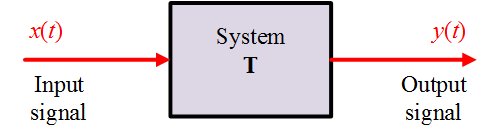A system is said to be memory less if its output for each value of the independent variable is dependent only on the input signal at that value of an independent variable.

• For example the system with description : y(t) = 5x(t) ( y(t) is the output signal corresponding to input signal x(t) ) is memoryless. In the physical world, a resistor can be considered to be a memoryless system (with voltage considered to be the input signal, current the output signal).
• By definition, a system that does not have this property is said to have memory.

How can we identify if a system has memory?

• For a memory less system, changing the input at any instant can change the output only at that instant. If in some cases, a change in input signal at some instant changes the output at some other instance, we can be sure that the system has memory.

Note: Consider a system whose output Y(t) depends on input X(t) as: Y(t) = X(t-5) + { X(t) - X(t-5) }

While at first glance, the system might appear to have memory, it does not. This brings us to the idea that given the description of a system, it need not at all be the most economical one. The same system may have more than one description.

Examples: Assume y[n] and y(t) are respectively outputs corresponding to input signals x[n] and x(t)

1. The identity system y(t) = x(t) is of-course Memory less.
2. System with description y[n] = x[n-5] has memory. The input at any "instant" depends on the input 5 "instants" earlier.
3. System with description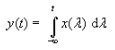also has memory. The output at any instant depends on all past and present inputs.

Question for Properties of Systems
Try yourself:Which of the following systems is memoryless?

Linearity

• Now we come to one of the most important and revealing properties systems may have - Linearity.
• Basically, the principle of linearity is equivalent to the principle of superposition, i.e. a system can be said to be linear if, for any two input signals, their linear combination yields as output the same linear combination of the corresponding output signals.

Definition: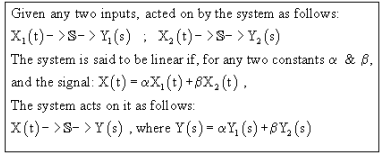(It is not necessary for the input and output signals to have the same independent variable for linearity to make sense. The definition for systems with input and/or output signal being discrete-time is similar.)

A capacitor, an inductor, a resistor or any combination of these are all linear systems if we consider the voltage applied across them as an input signal and the current through them as an output signal. This is because these simple passive circuit components follow the principle of superposition within their ranges of operation.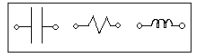Linearity can be thought of as consisting of two properties:

1. Additivity: A system is said to be additive if for any two input signals x1(t) and x2(t),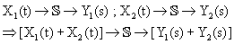i.e. the output corresponding to the sum of any two inputs is the sum of the two outputs.
2. Homogeneity (Scaling): A system is said to be homogenous if, for any input signal X(t),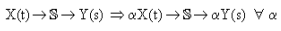i.e. scaling any input signal scales the output signal by the same factor.

To say a system is linear is equivalent to saying the system obeys both additivity and homogeneity.

(a) We shall first prove homogeneity and additivity imply linearity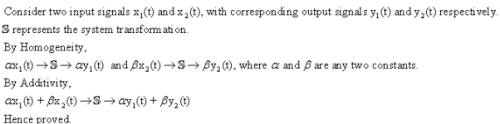(b) To prove linearity implies homogeneity and additivity.

This is easy; put both constants equal to 1 in the definition to get additivity; one of them to 0 to get homogeneity.

Additivity and Homogeneity are Independent Properties:

• We can prove this by finding examples of systems that are additive but not homogeneous, and vice versa.
• Again, y(t) is the response of the system to the input x(t).
• Example of a system that is additive but not homogeneous: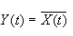[It is homogeneous for real constants but not complex ones - consider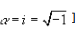Example of a system that is homogeneous but not additive: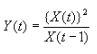[From this example can you generalize to a class of such systems?]

Examples: Assume y[n] and y(t) are respectively outputs corresponding to input signals x[n] and x(t)

1. System with description y(t) = t . x(t) is linear.
Consider any two input signals, x1(t) and x2(t), with corresponding outputs y1(t) and y2(t).
a and b are arbitrary constants. The output corresponding to a.x1(t) + b.x2(t) is = t (a.x1(t) + b.x2(t)) = t.a.x1(t) + t.b.x2(t), which is the same linear combination of y1(t) and y2(t).
Hence proved.
2. The system with description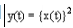is not linear.
See for yourself that the system is neither additive nor homogenous.
Show for yourself that systems with the following descriptions are linear: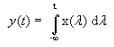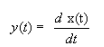Shift Invariance

• This is another important property applicable to systems with the same independent variable for the input and output signal. We shall first define the property for continuous-time systems and the definition for discrete-time systems will follow naturally.

Definition: Say, for a system, the input signal x(t) gives rise to an output signal y(t). If the input signal x(t - t0) gives rise to output y(t - t0), for every t0, and every possible input signal, we say the system is shift-invariant.

i.e. for every permissible x(t) and every t0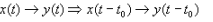In other words, for a shift-invariant system, shifting the input signal shifts the output signal by the same offset.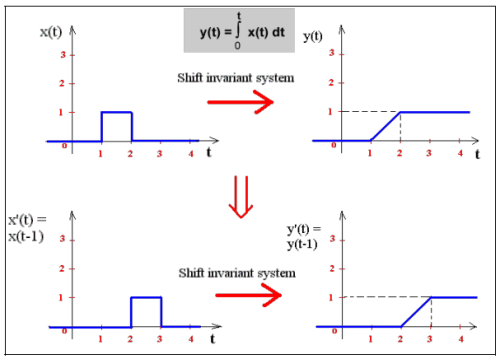Note this is not to be expected from every system. x(t) and x(t - t0) are different (related by a shift, but different) input signals and a system, which simply maps one set of signals to another, need not at all map x(t) and x(t - t0) to output signal also shift by t0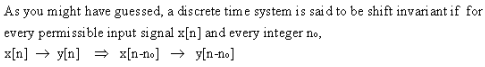A system that does not satisfy this property is said to be a shift variant.

Examples: Assume y[n] and y(t) are respectively outputs corresponding to input signals x[n] and x(t)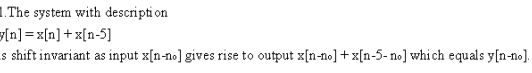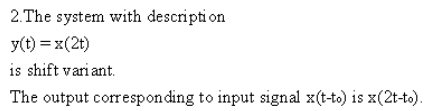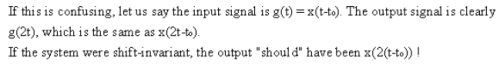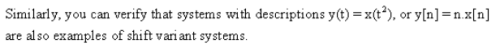Question for Properties of Systems
Try yourself:Which of the following systems is time invariant?

Stability

• Let us learn about one more important system property known as stability. Most of us are familiar with the word stability, which intuitively means resistance to change or displacement.
• Broadly speaking a stable system is one in which small inputs lead to predictable responses that do not diverge, i.e. are bounded. To get the qualitative idea let us consider the following physical example.

Example:

• Consider an ideal mechanical spring (elongation proportional to tension). If we consider tension in the spring as a function of time as the input signal and elongation as a function of time to be the output signal, it would appear intuitively that the system is stable. A small tension leads only to a finite elongation.
• There are various ideas/notions about stability not all of which are equivalent. We shall now introduce the notion of BIBO Stability, i.e. BOUNDED INPUT-BOUNDED OUTPUT STABILITY. Statement: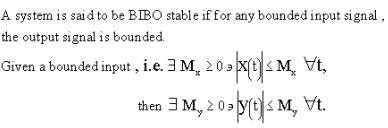Note:This should be true for all bound inputs x(t). It is not necessary for the input and output signal to have the same independent variable for this property to make sense. It is valid for continuous time, discrete time and hybrid systems.

Question for Properties of Systems
Try yourself:Which of the following systems is stable?

The document Properties of Systems - Notes | Study Signals and Systems - Electrical Engineering (EE) is a part of the Electrical Engineering (EE) Course Signals and Systems.
All you need of Electrical Engineering (EE) at this link: Electrical Engineering (EE)

## Signals and Systems

40 videos|44 docs|33 tests
 Use Code STAYHOME200 and get INR 200 additional OFF

## Signals and Systems

40 videos|44 docs|33 tests

Track your progress, build streaks, highlight & save important lessons and more!

,

,

,

,

,

,

,

,

,

,

,

,

,

,

,

,

,

,

,

,

,

;